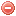# Description

The Fine fuel moisture code ($$FFMC$$) is one of the three fuel moisture code components of the Canadian forest fire weather index ($$FWI$$) system. The $$FFMC$$ represents the moisture content of litter and other cured fine fuels in a forest stand, in a layer of dry weight about 0.25 kg/m2, and assesses the relative ease of ignition and the flammability of fine fuels at mid-afternoon. It requires temperature, relative air humidity, wind speed and precipitation (at noon) as input data (Van Wagner 1987).

Like the two other fuel moisture codes of the $$FWI$$ (cf. $$DMC$$ and $$DC$$), the $$FFMC$$ comprises two phases: one for wetting by rain and one for drying.

As the $$FFMC$$ measures the moisture content in fine surface fuels, it is well appropriated for predicting fire occurrence (Van Wagner 1987).

# Formula

The $$FFMC$$ is calculated as follows (Van Wagner and Pickett 1985):

First, the previous day's $$FFMC$$ becomes $$FFMC_{t-1}$$.

Then, the fine fuel moisture content from the previous day $$m_{t-1}$$ has to be calculated:

$m_{t-1}=147.2\cdot\dfrac{101-FFMC_{t-1}}{59.5+FFMC_{t-1}}$

In case of rain (i.e. when $$P>0.5, cf. below), the fine fuel moisture content of the current day \(m_{r_t}$$ for wetting phases (which will become the new $$m_{t-1}$$) is calculated as follows:

$m_{r_t}= \begin{cases} m_{t-1}+42.5\cdot{P_f}\cdot\Big(e^{\frac{-100}{251-m_{t-1}}}\Big)\cdot\Big(1-e^{\frac{-6.93}{P_f}}\Big), & \mbox{for }m_{t-1}\leqslant{150} \\ & \\ m_{t-1}+42.5\cdot{P_f}\cdot\Big(e^{\frac{-100}{251-m_{t-1}}}\Big)\cdot\Big(1-e^{\frac{-6.93}{P_f}}\Big)+0.0015\cdot(m_{t-1}-150)^{2}\cdot{P_f}^{0.5}, & \mbox{for }m_{t-1}>150 \end{cases}$

where $$P_f$$ is effective rainfall [mm] and calculated as follows:

$P_f=P-0.5, \mbox{ for P}>0.5$

where $$P$$ [mm] is rainfall in open measured once daily at noon.

NB: if $$m_{r_t}$$ > 250, then $$m_{r_t}$$=250

Then, the fine fuel moisture content for drying phases where $$E_d$$ has to be calculated as follows:

$E_d=0.942\cdot{H_{12}^{0.679}}+11\cdot {e^\frac{H_{12}-100}{10}}+0.18\cdot(21.1-T_{12})\cdot(1-e^{-0.115\cdot H_{12}})$

where $$H_{12}$$ is relative air humidity [%] and $$T_{12}$$ air temperature [°C] at noon.

• If $$E_d$$ is smaller than $$m_{t-1}$$, then the log drying rate $$k_d$$ has to be calculated with the following equations:

$k_o=0.424\cdot\Bigg(1-\bigg(\dfrac{H_{12}}{100}\bigg)^{1.7}\Bigg)+0.0694\cdot U_{12}^{0.5}\cdot\Bigg(1-\bigg(\dfrac{H_{12}}{100}\bigg)^8\Bigg)$

$k_d=k_o\cdot{0.581\cdot e^{0.0365\cdot T_{12}}}$

where $$U_{12}$$ is wind speed [km/h] at noon.

Then, the fine fuel moisture content $$m$$ can be calculated as follows:

$m=E_d+(m_{t-1}-E_d)\cdot{10^{-k_d}}$

• If $$E_d is greater than \(m_{t-1}$$, then the fine fuel equilibrium moisture content for wetting phases $$E_w$$ has to be calculated instead:

$E_w=0.618\cdot {H_{12}^{0.753}}+10\cdot {e^\frac{H_{12}-100}{10}}+0.18\cdot(21.1-T_{12})\cdot(1-e^{-0.115\cdot H_{12}})$

• If $$E_w$$ is greater than $$m_{t-1}$$, then the log wetting rate $$k_w$$ has to be calculated with the following equations:

$k_1=0.424\cdot\Bigg(1-\bigg(\dfrac{100-H_{12}}{100}\bigg)^{1.7}\Bigg)+0.0694\cdot U_{12}^{0.5}\cdot\Bigg(1-\bigg(\dfrac{100-H_{12}}{100}\bigg)^8\Bigg)$

$k_w=k_1\cdot{0.581\cdot e^{0.0365\cdot T_{12}}}$

Then, the fine fuel moisture content $$m$$ can be calculated as follows:

$m_t=E_w-(E_w-m_{t-1})\cdot{10^{-k_w}}$

• If $$E_w\leqslant{m_{t-1}}\leqslant{E_d}$$, then $$m_t=m_{t-1}$$

Finally, the $$FFMC$$ is calculated as follows:

$FFMC_t=59.5\cdot\dfrac{250-m_t}{147.2+m_t}$

The $$FFMC$$ is supposed to be calculated on a daily basis. The meteorological data used for its calculation have to be recorded at noon (for fire danger prediction at about 4 pm).

The $$FFMC$$ calculation starts, in regions normally covered by snow in winter, on the third day after snow has essentially left the area. In regions where snow cover is not a significant feature, the calculation starts on the third successive day with noon temperature greater than 12 °C (Lawson and Armitage 2008). The starting value of the index has to be set to 85.

Original publications:
Van Wagner and Pickett (1985)
Van Wagner (1987)

Other publication:
Lawson and Armitage (2008)

### Bibliography SearchNo results!

No results were found. Are you sure you searched for a tag?

### Symbols

 Variable Description Unit $$T$$ air temperature °C $$T_{dew}$$ dew point temperature °C $$H$$ air humidity % $$P$$ rainfall mm $$U$$ windspeed m/s $$w$$ days since last rain (or rain above threshold) d $$rr$$ days with consecutive rain d $$\Delta t$$ time increment d $$\Delta{e}$$ vapor pressure deficit kPa $$e_s$$ saturation vapor pressure kPa $$e_a$$ actual vapor pressure kPa $$p_{atm}$$ atmospheric pressure kPa $$PET$$ potential evapotranspiration mm/d $$r$$ soil water reserve mm $$r_s$$ surface water reserve mm $$EMC$$ equilibrium moisture content % $$DF$$ drought factor - $$N$$ daylight hours hr $$D$$ weighted 24-hr average moisture condition hr $$\omega$$ sunset hour angle rad $$\delta$$ solar declination rad $$\varphi$$ latitude rad $$Cc$$ cloud cover Okta $$J$$ day of the year (1..365/366) - $$I$$ heat index - $$R_n$$ net radiation MJ⋅m-2⋅d-1 $$R_a$$ daily extraterrestrial radiation MJ⋅m-2⋅d-1 $$R_s$$ solar radiation MJ⋅m-2⋅d-1 $$R_{so}$$ clear-sky solar radiation MJ⋅m-2⋅d-1 $$R_{ns}$$ net shortwave radiation MJ⋅m-2⋅d-1 $$R_{nl}$$ net longwave radiation MJ⋅m-2⋅d-1 $$\lambda$$ latent heat of vaporization MJ/kg $$z$$ elevation m a.s.l. $$d_r$$ inverse relative distance Earth-Sun - $$\alpha$$ albedo or canopy reflection coefficient - $$\Delta$$ slope of the saturation vapor pressure curve kPa/°C $$Cc$$ cloud cover eights $$ROS$$ rate of spread m/h $$RSF$$ rate of spread factor - $$WF$$ wind factor - $$WRF$$ water reserve factor - $$FH$$ false relative humidity - $$FAF$$ fuel availability factor - $$PC$$ phenological coefficient -

 Suffix Description $$-$$ mean / daily value $$_{max}$$ maximum value $$_{min}$$ minimum value $$_{12}$$ value at 12:00 $$_{13}$$ value at 13:00 $$_{15}$$ value at 15:00 $$_{m}$$ montly value $$_{y}$$ yearly value $$_{f/a}$$ value at fuel-atmosphere interface $$_{dur}$$ duration $$_{soil}$$ value at soil level

 Constant Description $$e$$ Euler's number $$\gamma$$ psychrometric constant $$G_{SC}$$ solar constant $$\sigma$$ Stefan-Bolzmann constant